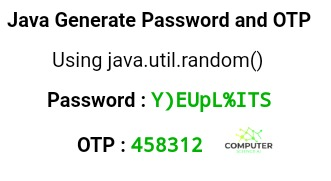# Java Generate Password and OTP

Generate password and otp in java. To generate a strong password containing upper case, lower case, numbers and special symbols we are going to use the `random()` method of `java.util.random` class. For generating a 6 digit OTP also we are going to do the same. Examples of generating password and otp in java is given below.## OTP Generation in Java

### How do you generate a 6 digit OTP (One Time Password) in java?

Generating a OTP is very much like generating a password but only difference is that it is numeric only. And moreover generating a six (6) digit OTP is just having length of OTP as 6 digits wide.

Most of the websites need otp generation nowadays for various reasons. One of the main reason is that if you forget your password you can just put your mobile number and get otp and set new password.

So if you are such website owner and need the java code to do the work automatically here is the java code to generate otp and password in just seconds.

### Java Program to Generate a 6 Digit OTP

``````import java.util.*;

public class Generate_OTP_Java {

// static method to generate OTP
static char[] OTP_Generate(int len)
{
System.out.println("Generating OTP using random() : ");
System.out.print("You OTP is : ");

// Using numeric values
String numbers = "0123456789";

// Using random method
Random rdm_method = new Random();

// to store the OTP
char[] OTP = new char[len];

for(int i = 0; i < len; i++)
{
// Use of charAt() method to get character value
// Use of nextInt() as it is scanning the value as int
OTP [i] = numbers.charAt(rdm_method.nextInt(numbers.length()));
}
return OTP;
}

public static void main(String[] args)
{
// set the desired length to generate a OTP of
int OTPLength = 6;
System.out.println(OTP_Generate(OTPLength));
}
}

/* Output of above code:-

Generating OTP using random() :
You OTP is : 458312

*/``````

``````import java.util.*;

{
public static void main(String[] args) {
// let the length of password be 10 characters
int length = 10;
}

// static method to generate password
// it is static so that we can
// execute it without creating any objects
{
System.out.println("Generating New Password using random() : ");

// Our password must contain all
// type of characters lower, upper and special case and numbers
String lowerChars = "abcdefghijklmnopqrstuvwxyz";
String capitalChars = "ABCDEFGHIJKLMNOPQRSTUVWXYZ";
String symbols = "!@#\$%^&*_=+-/.?<>)";
String numbers = "0123456789";

String values = lowerChars + capitalChars +
numbers + symbols;

// Using random() method
Random rdm_method = new Random();

// to store our final password

for(int i = 0; i < len; i++)
{
// Use of charAt() method to get character value
// Use of nextInt() as we are scanning the value as int

}